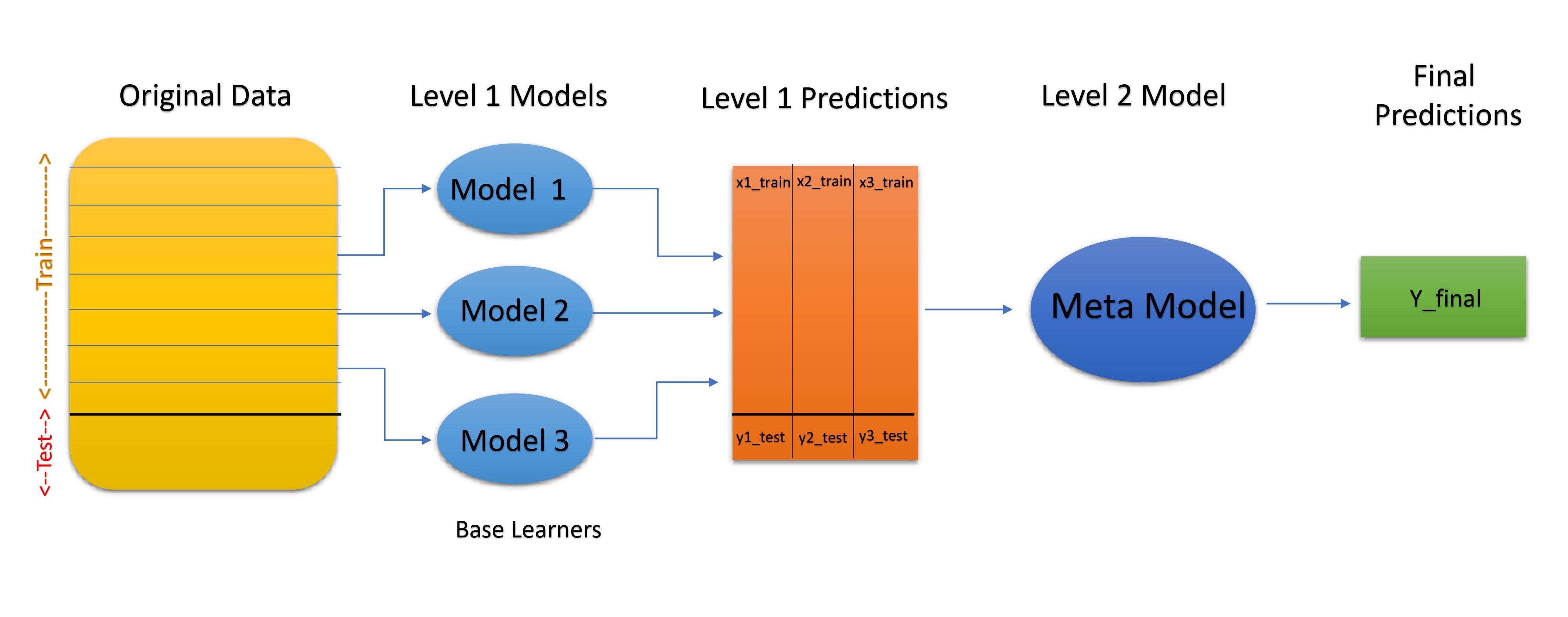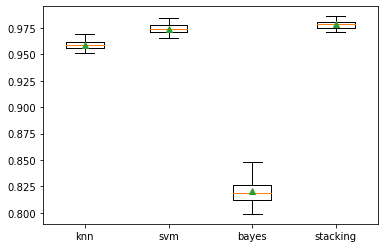×

Search anything:

# Stacking in Machine Learning

#### Machine Learning (ML)Get this book -> Problems on Array: For Interviews and Competitive Programming

"When there is Unity, there is always victory"

Ensemble modeling is a powerful way to improve the performance of your model. It is a machine learning paradigm where multiple models are trained to solve the same problem and combined to get better results. It is also an art of combining a diverse set of learners together to improvise on the stability and predictive power of the model.

Most common types of Ensemble Methods:

1. Bagging
2. Boosting
3. Stacking

• What is Stacking?
• The general architecture of Stacking
• Steps to implement Stacking
• Basic Code implementation using Sckit-Learn
• How Stacking differs from Bagging and Boosting

### What is Stacking?

Stacking (a.k.a Stack Generalization) is an ensemble technique that uses meta-learning for generating predictions. It can harness the capabilities of well-performing as well as weakly-performing models on a classification or regression task and make predictions with better performance than any other single model in the ensemble.

It is an extended form of the Model Averaging Ensemble technique, where multiple sub-models contribute equally or according to their performance weights to a combined prediction. In Stacking, an entirely new model to trained to combine the contributions from each submodel and produce the best predictions. This final model is said to be stacked on top of the others, hence the name.

### The Architecture of Stacking:• Original Data - The original split is split into n-folds
• Base Models - Level 1 individual Models
• Level 1 Predictions - Predictions generated by base models on original data
• Level 2 Model - Meta-Learner, the model which combines the Level 1 predictions to generate best final Predictions

Stacking can have more than one level of base learners.

### Steps of Implementation

The following steps are involved in implementation:

1. The Original Train data is split into n-folds using the RepeatedStratifiedKFold.
2. Then the base learner (Model 1) is fitted on the first n-1 folds and predictions are made for the nth part.
3. This prediction is added to the x1_train list.
4. Steps 2 & 3 are repeated for the rest of the n-1 parts and we obtain x1_train array of size n

where, x1_train[i] is the prediction on (i+1)th part, when the model 1 is fitted on 1,2...,i-1,i+1...n parts

1. Now, train the model on all the n parts and make predictions for test data. Store this prediction in y1_test.
2. Similarly, we obtain x2_train, y2_test, x3_train and y3_test by using Model 2 and 3 for training respectively to obtain Level 2 predictions.
3. Now we train a Meta Learner on Level 1 Predictions (using these predictions as features for the model).
4. The Meta learner is now used to make predictions on test data.

### Code Implementation

We can implement stacking using StackingClassifier provided by scikit-learn. It is available in version 0.22 of the library and higher.

Here is the basic code implementation:
``````# Import Libraries
from sklearn.datasets import make_classification
from sklearn.model_selection import cross_val_score
from sklearn.model_selection import RepeatedStratifiedKFold
from sklearn.linear_model import LogisticRegression
from sklearn.neighbors import KNeighborsClassifier
from sklearn.tree import DecisionTreeClassifier
from sklearn.svm import SVC
from sklearn.naive_bayes import GaussianNB
from sklearn.ensemble import StackingClassifier
from matplotlib import pyplot

#function to create a random dataset using sklearn's make_classification
def get_dataset():
X, y = make_classification(n_samples=10000, n_features=20, n_informative=15, n_redundant=5, random_state=1)
return X, y

# evaluate a give model using cross-validation
def evaluate_model(model, X, y):
cv = RepeatedStratifiedKFold(n_splits=10, n_repeats=3, random_state=1)
scores = cross_val_score(model, X, y, scoring='accuracy', cv=cv, n_jobs=-1, error_score='raise')
return scores

X,y=get_dataset()

level1 = list()                                    # stores the level1 base models
level1.append(('knn', KNeighborsClassifier()))     # model1
level1.append(('svm', SVC()))                      # model2
level1.append(('bayes', GaussianNB()))             # model3

meta_Learner = LogisticRegression()                # define meta learner model

stacked_model = StackingClassifier(estimators=level1, final_estimator=meta_Learner, cv=4)     #defining the StackingClassifier

# get the base models
models = dict()
models['knn'] = KNeighborsClassifier()
models['svm'] = SVC()
models['bayes'] = GaussianNB()
models['stacking'] = stacked_model

# evaluate the models and store results
results, names = list(), list()
print('Base models individual performances')
for name, model in models.items():
scores = evaluate_model(model, X, y)
results.append(scores)
names.append(name)
if name=='stacking':
print(' ')
print('Stacking Classifier performance')
print('%s %.3f (%.3f)' % (name, mean(scores), std(scores)))

# plot model performance
pyplot.boxplot(results, labels=names, showmeans=True)
pyplot.show()

``````
``````Output:

Base models individual performances
knn 0.959 (0.004)
svm 0.974 (0.005)
bayes 0.821 (0.011)

Stacking Classifier performance
stacking 0.978 (0.004)
``````It can be seen that stacked ensemble model give the highest accuracy amongst all the models.

### Difference between Bagging, Boosting and Stacking

Now, let's see how stacking differs from bagging and boosting.

• Basic Definition

• Bagging: Generally, it is used in order to reduce the variance of a model. Here, we create several randomly selected overlapping subsets of data from the training sampple. Each of subset is used for training the model. In this way we get ensemble of different models.
• Boosting: Boosting uses multiple predictors. It is a sequential ensemble method that builds strong predictive models by decreasing bias error. In this technique, data samples are weighted, such that the upcoming model focuses more on the important(generally misclassified) data points. During training, weights are also allocated to models. So models which perform better have higher weights.
• Stacking: Similar to boosting, we also apply several models to your original data. The difference here is, we don't allocate weights, rather we introduce a meta-level i.e. using another model/approach to estimate the input together with outputs of every model to estimate the weights of individual models.
• Working Principle

• Bagging: Here we build several models independently and then average their predictions o get the final predictions
• Boosting: In boosting we develop models sequentially and try to reduce bias upon each iteration
• Stacking: The predictions of base learners is used as a feature to obtain the fianl prediction by the meta-learner which is stacked upon all the base learners
Boosting Bagging Stacking
Splitting of data random weighted preference n-folds
Target reduce variance reduce bias reduce bias and variance
Implemented in... random subspace gradient boosting blending
Method to combine predictions weighted average weighted majority vote logistic regression

For the final part to check your learning, try to answer the following question:

### TRUE or FALSE

#### The meta learner is used to extract features from the original data

True
False
Base Learners are used for feature extraction and not the meta learner. Hence the answer is FALSE.

Stacking has been proved to be highly effective in kaggle competitions. So next time when you participate in any competition, you will have a powerful weapon in your hand ;)

Wanna dig deeper? Look at the give links: